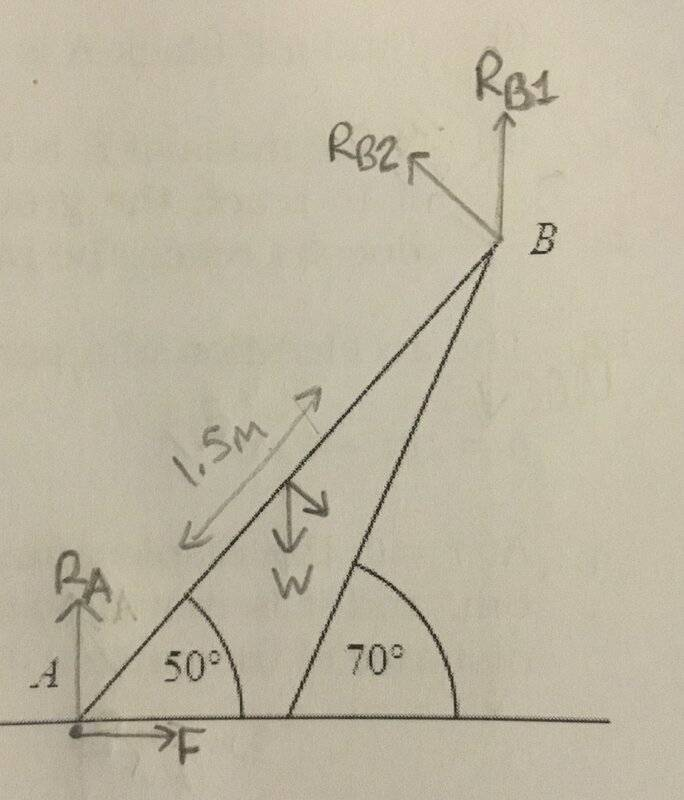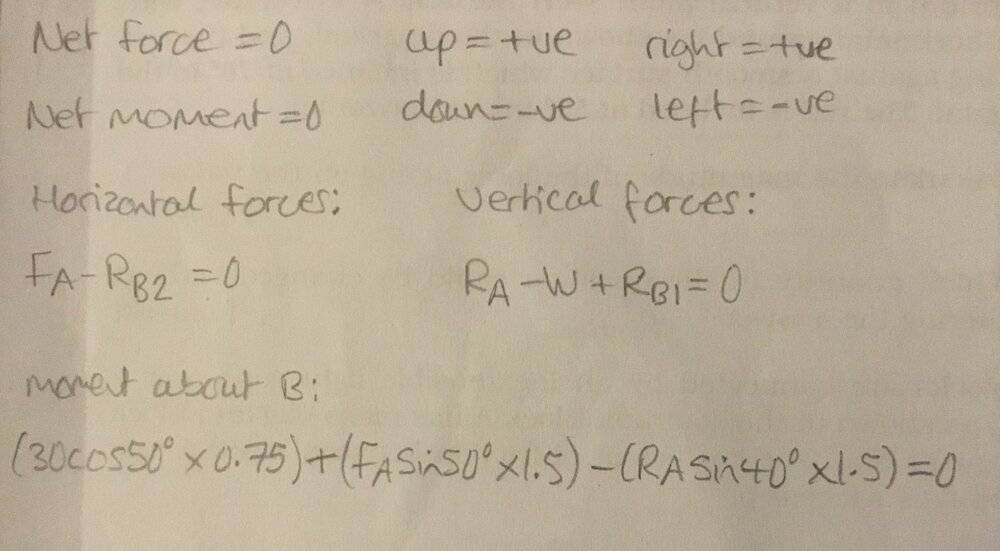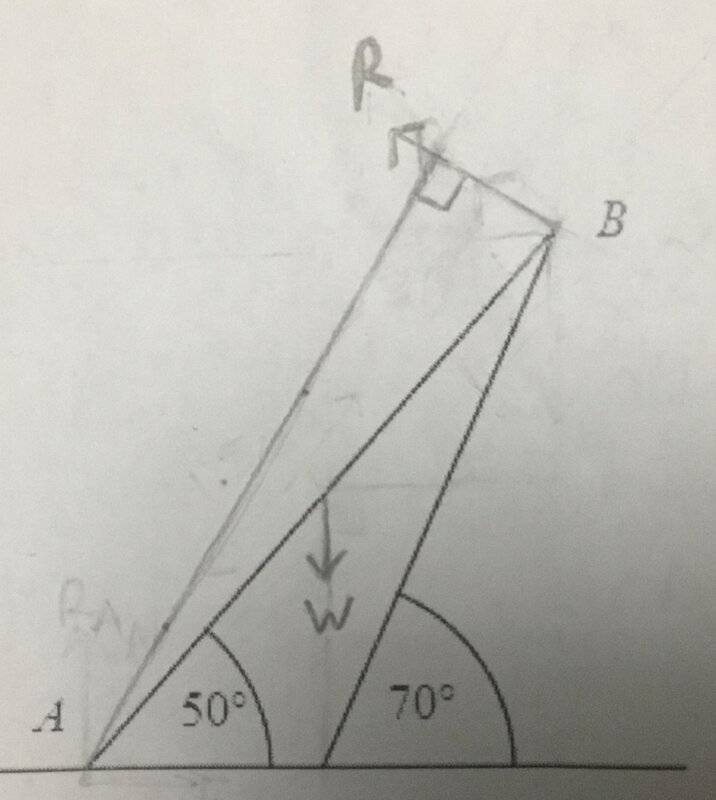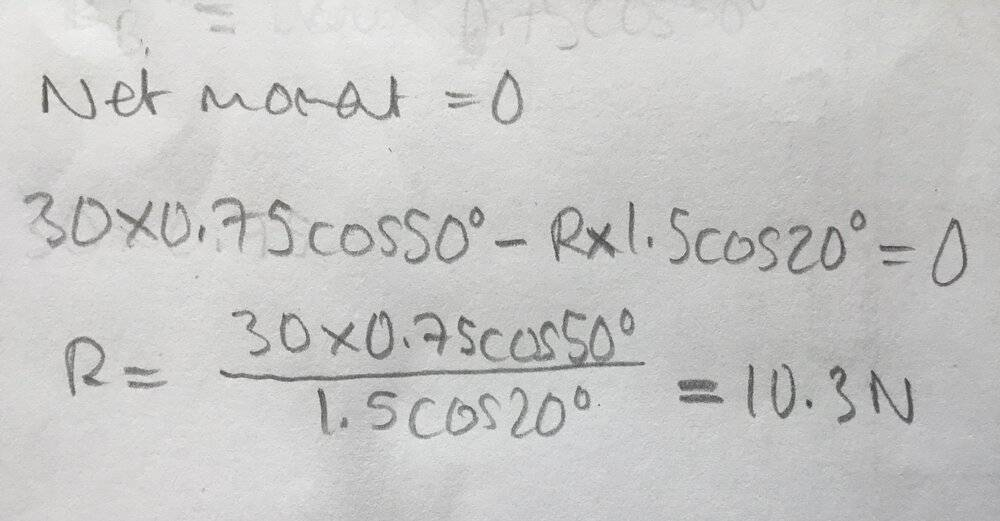# Force at the end of a Rod at rest on an inclined surface

• so_gr_lo

#### so_gr_lo

Homework Statement
End B of uniform rod of length 1.5 weight 30N rests against smooth surface inclined at 70°. Other end, A, of rod rests on rough ground at 50°. Find the magnitude of the force acting at B.
Relevant Equations
moment = F x d
Question diagram, attempt at solution belowI need to cancel some of the terms in the moment equation but a not sure which ones to start with. I don’t know μ so can not calculate FA, so should probably substitute FA = RB2.

I find your diagram and equations a bit confusing. Realize that at B, the force exerted by the surface on the rod must be perpendicular to the surface (because the surface is smooth).

Homework Statement:: End B of uniform rod of length 1.5 weight 30N rests against smooth surface inclined at 70°. Other end, A, of rod rests on rough ground at 50°. Find the magnitude of the force acting at B.
Relevant Equations:: moment = F x d

Question diagram, attempt at solution below
View attachment 274416
View attachment 274417
moment = F x d is only true if F and d are perpendicular, i.e. if d is the length of the 'lever-arm' as shown here: https://slideplayer.com/slide/14635...Scalar+Analysis+Mo+=+F+d+Mo+=+F+(r+sin+θ).jpg

One arrow from the rod's middle is the weight (W). No idea what the other arrow is.

You have marked the length incorrectly. The arrow heads should be level with the ends of the rod.

No idea what ##R_{B1}## is. But ##R_{B2}## is perpendicular to the (tilted) wall and there is no friction at B. So ##R_{B2}## is the required force.

There is no need consider horizontal/vertical forces. Take moments about point A. The clockwise moment produced by W equals the anticlockwise moment produced by ##R_{B2}##. That gives you a single equation from which to work out ##R_{B2}##.

You just need to use some simple trig’ to work out the lever -arm distances (d) for W and for ##R_{B2}##.

•Delta2 and Chestermiller
moment = F x d is only true if F and d are perpendicular, i.e. if d is the length of the 'lever-arm' as shown here: https://slideplayer.com/slide/14635159/90/images/9/Calculating+moment+Scalar+Analysis+Mo+=+F+d+Mo+=+F+(r+sin+θ).jpg

One arrow from the rod's middle is the weight (W). No idea what the other arrow is.

You have marked the length incorrectly. The arrow heads should be level with the ends of the rod.

No idea what ##R_{B1}## is. But ##R_{B2}## is perpendicular to the (tilted) wall and there is no friction at B. So ##R_{B2}## is the required force.

There is no need consider horizontal/vertical forces. Take moments about point A. The clockwise moment produced by W equals the anticlockwise moment produced by ##R_{B2}##. That gives you a single equation from which to work out ##R_{B2}##.

You just need to use some simple trig’ to work out the lever -arm distances (d) for W and for ##R_{B2}##.
Apart from the way RB2 is drawn (seems it it supposed to be horizontal), everything looks correct so far. Also, it would be clearer if the surface were drawn to extend beyond the rod.
But there are only three equations and four unknowns. As @Doc Al posted, the unused fact is that the angled surface is smooth.

@so_gr_lo, a useful trick with such problems is to look for forces that you neither know nor need to know. By carefully choosing the axis to take moments about you can often eliminate these and get straight to the key equation.

My initial attempt at the solution was based on a tutorial for a similar question, however this question had a rough wall, so I included unnecessary forces.

if I take moments about A I can ignore RA and F. Below is my second attempt, but I am not sure if the line I have drawn is the correct perpendicular distance from R to A.My initial attempt at the solution was based on a tutorial for a similar question, however this question had a rough wall, so I included unnecessary forces.

if I take moments about A I can ignore RA and F. Below is my second attempt, but I am not sure if the line I have drawn is the correct perpendicular distance from R to A.

View attachment 274465
You know R is perpendicular to the surface, and you want to draw a line perpendicular to R, so...

So the length of the surface is the perpendicular distance between R and A?

So the length of the surface is the perpendicular distance between R and A?
No, I wanted you to think about the direction of that perpendicular distance in relation to the plane of the surface.

The perpendicular distance is parallel to the surface

The perpendicular distance is parallel to the surface
Right. So if that distance meets the line of action of R at C, what is angle CAB?

•Steve4Physics
20°

•Steve4Physics
20°
Can I add that if the bottom of the wall is P, then ∠PBC = 90° and the lever arm (AC) is parallel to PB. Also ∠PBA = 20°. An accurate diagram can often make it much easier to see what's going on.

So for R I get•Lnewqban
Finally. Thanks for the help.